First, let us distinguish several similar terms. What is the different between quadratic function from quadratic equation and quadratic formula?

Function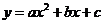is called quadratic function when the three parameters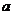,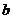and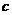are real numbers. Quadratic function is more general function than a linear function because when the value of parameter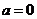, then the quadratic function degenerate becomes a linear function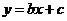(linear means straight line geometrically). When the function value is set to be equal to zero (i.e.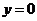), the quadratic function is called quadratic equation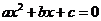.

The formula to find the roots of quadratic equation is called quadratic formula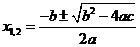or often called ABC formula because it involves three parameters,and.

We will explore more the three quadratic terms above. First, we discuss about the characteristics of quadratic function.

Geometrically, quadratic functionproduces a parabola curve when we plot in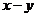plane. The shape and characteristics of the quadratic function is closely related to the parabola and depends on the value of the parameters.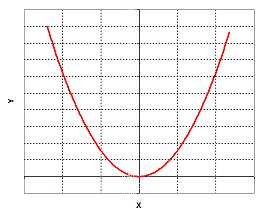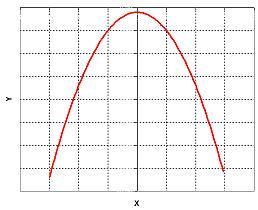Smiling parabola when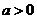Sad parabola when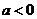• The parameter attached to the quadratic term () is called quadratic parameter or shape parameter because it influences the shape of the parabola. When the quadratic parameter is positive (), the parabola is opened upward, look like a smile (it is sometimes called concave up ). The lowest or highest point on the parabola is called extreme point . For a smiling parabola, the extreme point is at minimum.
• When the quadratic parameter is negative (), the parabola is opened downward, look like a sad mouth (it is sometimes called concave down ). The extreme point of sad parabola is at maximum.
• Of course, if the quadratic parameter is zero (), the quadratic function becomes linear function and has no extreme point.
• The second parameter () is the gradient of linear function degenerated from quadratic function.
• If we input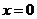to quadratic function, we get an intercept or a crossing point (at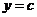) between parabola curve and vertical axis. Thus, the third parameter () determine the intercept point. If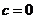then the parabola will pass the origin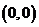.
• The extreme point is at coordinate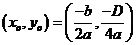where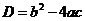is called discriminant of quadratic equation. We will discuss more about discriminant in the next section . The vertical line passing the extreme point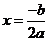divides the parabola symmetrically into two parts. Therefore, this vertical line is called axis of symmetry .

Quadratic function may appear in several forms. Below are the forms that are typically found in many applications.

1. Polynomial form. This is the general form that we are using throughout the tutorial
2. Standard form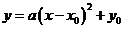whereis the extreme point (maximum or minimum point) where. We will discuss the application example of extreme point in later section . Inputting the coordinate of extreme point to the standard form yield general polynomial form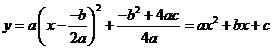3. Factored form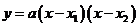where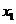and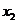are the roots of the quadratic equation where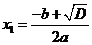and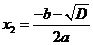. Inputting the roots into the factored form produces general polynomial form.